# PSAT Math : How to find the length of the diagonal of a rhombus

## Example Questions

### Example Question #282 : Plane Geometry

In Rhombus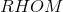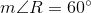. If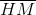is constructed, which of the following is true about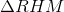?is obtuse and isosceles, but not equilateralis acute and scaleneobtuse and scaleneis acute and isosceles, but not equilateralis acute and equilateralis acute and equilateral

Explanation:

The figure referenced is below.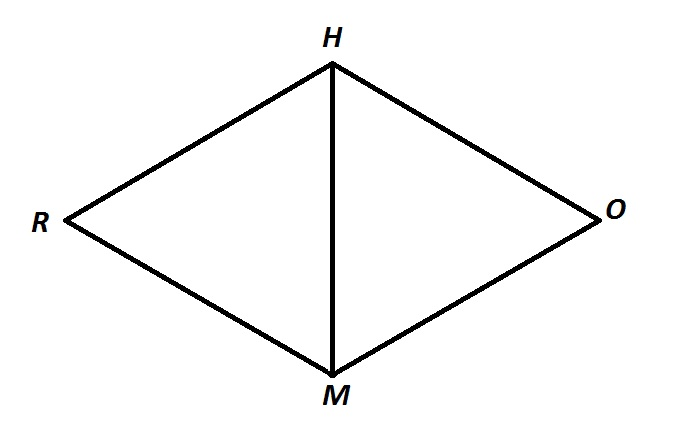Consecutive angles of a rhombus are supplementary - as they are with all parallelograms - so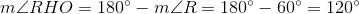A diagonal of a rhombus bisects its angles, so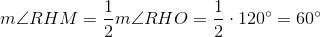A similar argument proves that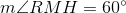.

Since all three angles ofmeasure, the triangle is acute. It is also equiangular, and, subsequently, equilateral.